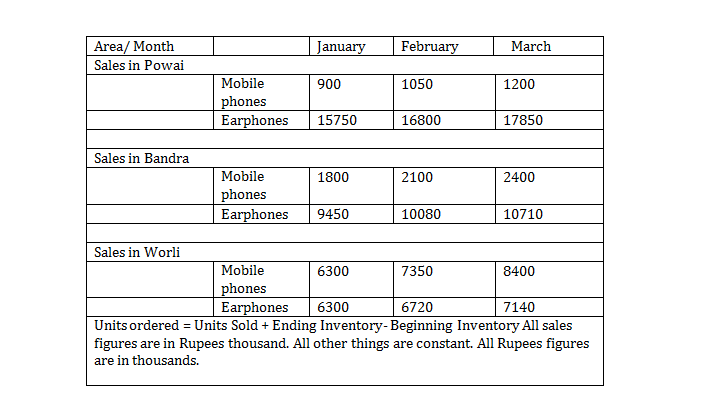Answer the question no. 1 to 5 on the basis of the data given below.Question 1. In the period from January to March, Chroma Electronics sold 3150 unit of Mobile phones, having started with a beginning inventory of 2520 units and ending with an inventory of 2880. What was value of order placed (Rupees in thousands) by Chroma Electronics during the three months period? [Profits are 25% of cost price, uniformly].
A. 28080
B. 2808
C. 26325
D. 22320
E. 25200

Question 2. What was the total value of surcharge paid – at the rate of 14% of sales value – by Chroma Electronics, over the period of three months?
A. Cannot be calculated
B. 18548
C. 18425
D. 18522
E. 18485

Question 3. 10% of sales price of Earphones and 20% of sales price of Mobile phones contribute to the profits  of Chroma Electronics. How much profit did the company earn in the month of January from Powai and Worli from the two products?
A. 513
B. 4410
C. 3645
D. 5230
E. 5350

Question 4. In the period from January to March, consider that Chroma Electronics ordered 7560 units of Earphones for all three areas put together. What was unit sales price of Earphone during the period? The ending inventory was 6120 units and the beginning inventory stood at 5760.
A. 14.65
B. 14.80
C. 13.00
D. 13.60
E. 14.00

Question 5. For Chroma Electronics beginning inventory was 720 for Mobile phones and 1800 for Earphones and ending inventory was 840 for Mobile phones and 1920 for Earphone in the month of January. How many units of Mobile phones and Earphones did Chroma Electronics order for the month of January? Additional data: In the month of February, 1050 units of Mobile phones and 2400 units of Earphones were sold in all three areas put together.
A. 1020, 2270
B. 1020, 2370
C. 2270, 1030
D. 1030, 2370
E. 1020, 2280

Units ordered = Units sold + Ending inventory – Beginning inventory

= 3150 + 2880 – 2520 = 351 0

Total sales of Mobile phones =

900 + 1800 + 6300 + 1050 +2100 + 7350 + 1200 + 2400 + 8400 = Rs.31,500

∴Sales price per unit of Mobile phones =  = Rs.10

Cost price per unit of Mobile phones =  = Rs.8

Total value of order placed = 3510 × 8 = Rs.28,080.

From the above table we add all the sales value, column wise

= 40500 + 47700 + 44100 = 132300

14% of 132,300 = 18522.

Hence, (D) is the correct option.

For Powai and Worli, Earphone sale value = 22050

And Mobile phones sales value = 7200

Profit = 10% × 22050 + 20% × 7200 = 3645

Given,

Units ordered = Units sold + Ending Inventory – Beginning Inventory

7560 = units sold + 6120 – 5760i.e units sold = 7200

Earphones sold (in rupees thousand) = 100800

∴ unit sales price = =14

In a month of February, 1050 units of Mobile phones and 2400 unitsof Earphones were sold in all 3 areas.

S.P per unit of Mobile phones = =Rs. 10

S.P of Earphone per unit = = Rs. 14

This Price per unit is from a month of January. Number of units of Mobile phones sold in the month of January

Number of units of Earphones sold in the month of January =

We know that, Number of units ordered = Units sold + Ending Inventory – Beginning Inventory

For Mobile phones, units ordered = 900 + 840 – 720 = 1020

For Earphone, units ordered = 2250 + 1920 – 1800 = 2370